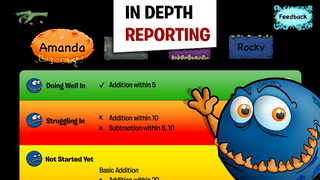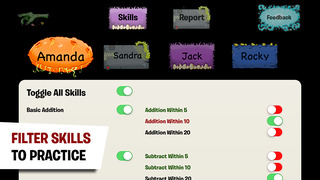## Monster Math - Free Fun Math Game - Learning Addition, Subtraction, Multiplication and Division helps you practice Grade 1,2,3,4 Math - Lumos Educational App Store41
Price - Free
\$0

#### OVERVIEW:

Monster Math - Free Fun Math Game - Learning Addition, Subtraction, Multiplication and Division is a free educational mobile app By Makkajai Edu Tech Private Limited.It helps students in grades 1,2,3,4 practice the following standards 3.OA.1,4.OA.1,.

This page not only allows students and teachers download Monster Math - Free Fun Math Game - Learning Addition, Subtraction, Multiplication and Division but also find engaging Sample Questions, Videos, Pins, Worksheets, Books related to the following topics.

1. 3.OA.1 : Interpret products of whole numbers, e.g., interpret 5 .

2. 4.OA.1 : Interpret a multiplication equation as a comparison, e.g., interpret 35 = 5 x 7 as a statement that 35 is 5 times as many as 7 and 7 times as many as 5. Represent verbal statements of multiplicative comparisons as multiplication equations..

1
2
3
4

#### STANDARDS:

3.OA.1
4.OA.1

Developer: Makkajai Edu Tech Private Limited

Software Version: 5.3

Category: Education

Release Date: 2014-11-21T13:39:18Z### RELATED APPSEdSearch WebSearch